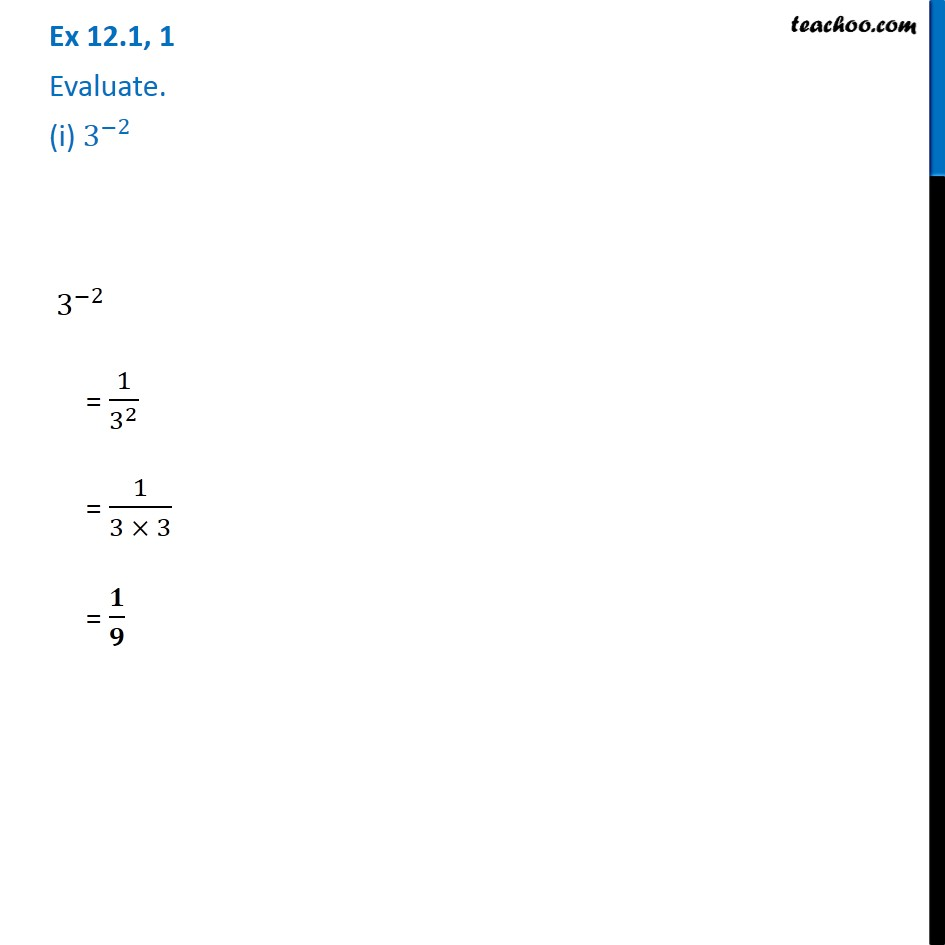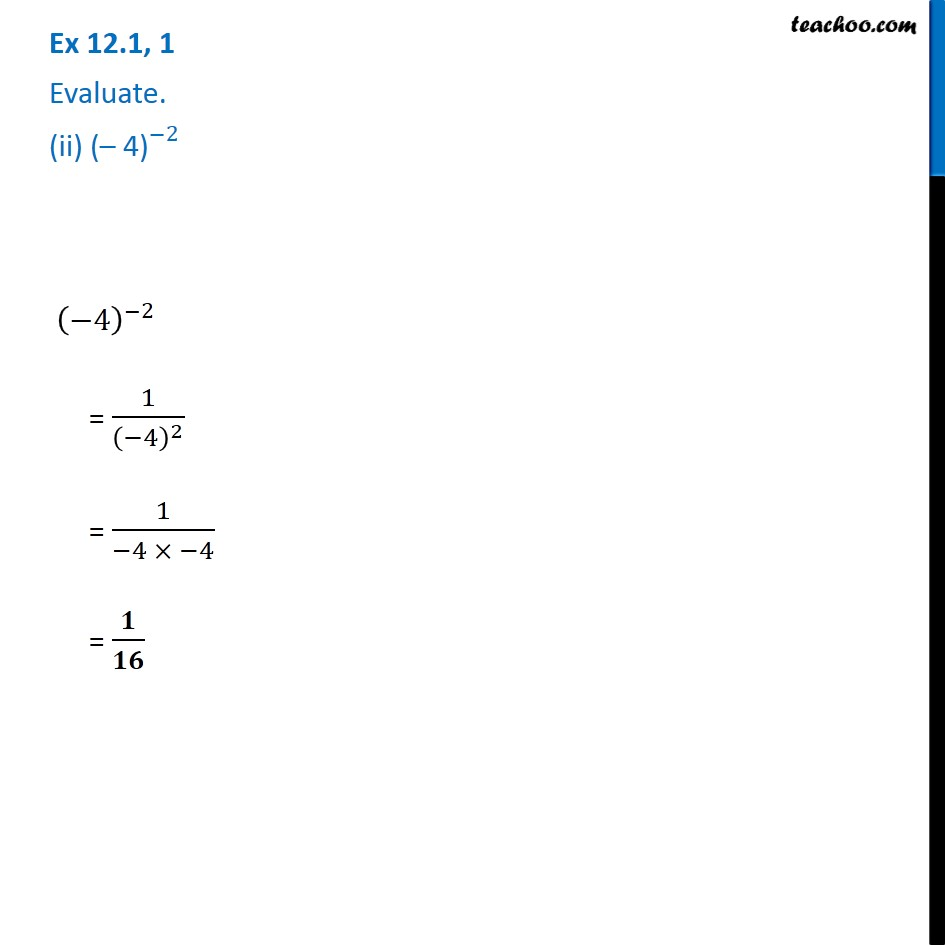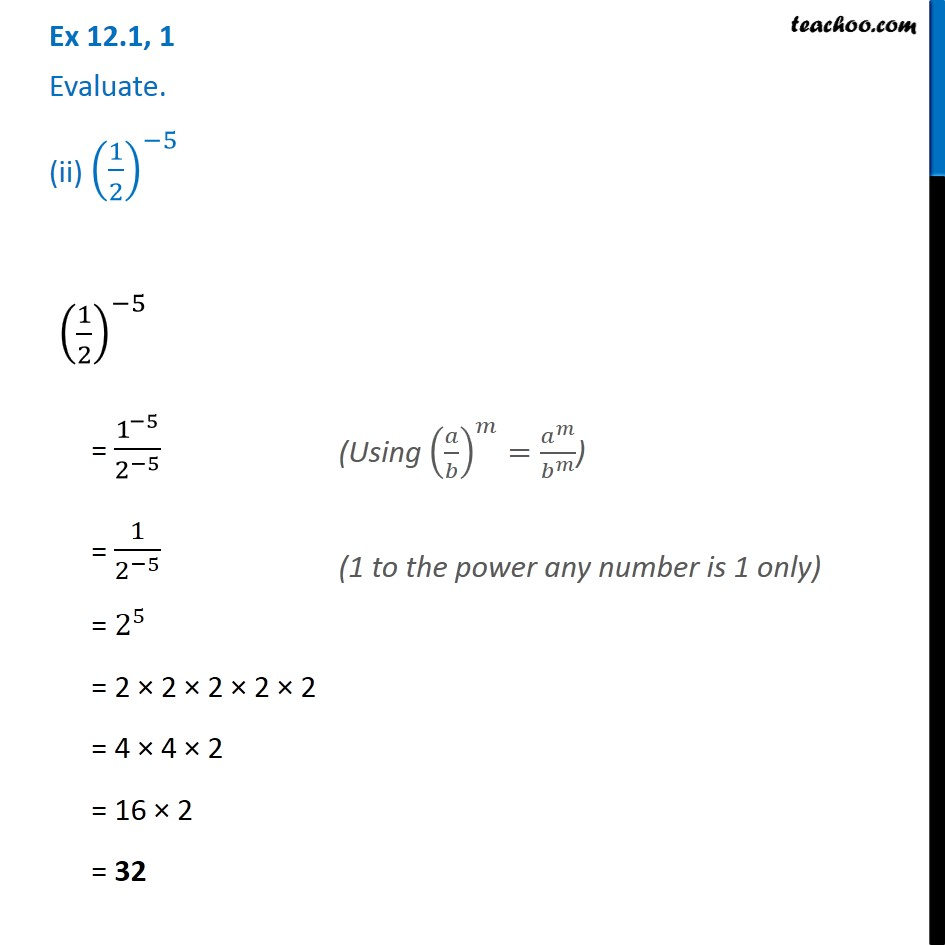1. Chapter 12 Class 8 Exponents and Powers
2. Serial order wise
3. Ex 12.1

Transcript

Ex 12.1, 1 Evaluate. (i) 3^(−2) 3^(−2) = 1/3^2 = 1/(3 × 3) = 𝟏/𝟗 Ex 12.1, 1 Evaluate. (ii) 〖"(– 4)" 〗^(−2) (−4)^(−2) = 1/(−4)^2 = 1/(−4 × −4) = 𝟏/𝟏𝟔 Ex 12.1, 1 Evaluate. (ii) (1/2)^(−5) (1/2)^(−5) = 1^(−5)/2^(−5) = 1/2^(−5) = 2^5 = 2 × 2 × 2 × 2 × 2 = 4 × 4 × 2 = 16 × 2 = 32 (Using (𝑎/𝑏)^𝑚=𝑎^𝑚/𝑏^𝑚 ) (1 to the power any number is 1 only)

Ex 12.1